• 向量积模的几何意义是
千次阅读
2021-01-10 20:14:06

### 向量叉乘的几何意义

对于两个2维向量:
a ⃗ = ( x 1 , y 1 ) b ⃗ = ( x 2 , y 2 ) \begin{aligned} \vec{a} &= (x1,y1) \\ \vec{b} &= (x2,y2) \end{aligned}

叉乘定义:
｜ a ⃗ × b ⃗ ｜ = x 1 y 2 − x 2 y 1 ｜\vec{a} \times \vec{b}｜ = x_1y_2-x_2y_1

#### 计算面积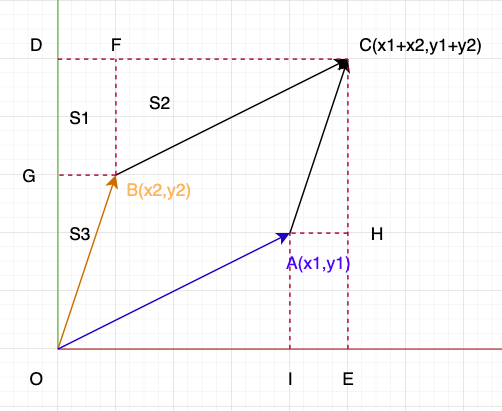四边形ODCE面积:
S = ( x 1 + x 2 ) ( y 1 + y 2 ) S = (x_1+x_2)(y_1+y_2)

四边形GDFB面积:
S 1 = x 2 y 1 S_{1} = x_2y_1

三角形BFC面积:
S 2 = 0.5 ( x 1 y 1 ) S_{2}=0.5(x_1y_1)

三角形OGB面积:
S 3 = 0.5 ( x 2 y 2 ) S_{3}=0.5(x_2y_2)

平行四边形OGCA面积:
S 平 行 四 边 形 = S − 2 S 1 − 2 S 2 − 2 S 3 = ( x 1 + x 2 ) ( y 1 + y 2 ) − 2 x 2 y 1 − x 1 y 1 − x 2 y 2 = x 1 y 1 + x 2 y 1 + x 1 y 2 + x 2 y 2 − 2 x 2 y 1 − x 1 y 1 − x 2 y 2 = x 1 y 2 − x 2 y 1 \begin{aligned} S_{平行四边形} &= S-2S_1-2S_2-2S_3 \\ &= (x_1+x_2)(y_1+y_2) - 2x_2y_1 - x_1y_1 - x_2y_2 \\ &= x_1y_1+x_2y_1 + x_1y_2+x_2y_2 - 2x_2y_1 - x_1y_1 - x_2y_2 \\ &= x_1y_2 - x_2y_1 \end{aligned}

#### 结论:

向量叉乘的模表示的是所围成平行四边形的面积。

更多相关内容
• 向量叉乘定义，行列式和面积关系

目的：在传统的向量叉乘计算中，常常遇到叉乘。定义为向量。其这个向量方向满足右手定则。它的模大小，一般被忽略。因此推测一下。

向量叉乘定义：
外积（英语：Cross product）又称向量积（英语：Vector product），是对三维空间中的两个向量的二元运算，用符号： × \times 表示。可以定义为：
a → × b → = c →      ( 1 ) \overrightarrow{a} \times \overrightarrow{b} = \overrightarrow{c} \space \space \space \space(1)
假设两个向量 a → × b → \overrightarrow{a} \times \overrightarrow{b} 外积,它的方向为 c → \overrightarrow{c} 。其方向由右手定则决定。模长等于这两个向量边的平行四边形的面积。
它的定义也可以写成：
a → × b → = ∣ a → ∣ ∣ b → ∣ s i n ( θ ) n →      ( 2 ) \overrightarrow{a} \times \overrightarrow{b} = |\overrightarrow{a}||\overrightarrow{b}|sin(\theta)\overrightarrow{n} \space \space \space \space(2)
其中 θ \theta 为两个向量的夹角 0 ≤ θ ≤ 180 0\le \theta \le 180 ∣ a → ∣ ∣ b → ∣ |\overrightarrow{a}||\overrightarrow{b}| 分别为两个向量 a → b → \overrightarrow{a} \overrightarrow{b} 的模长。 n → \overrightarrow{n} 为垂直于 a → b → \overrightarrow{a} \overrightarrow{b} 所在平面的法向量，且它满足右手定则。如下图：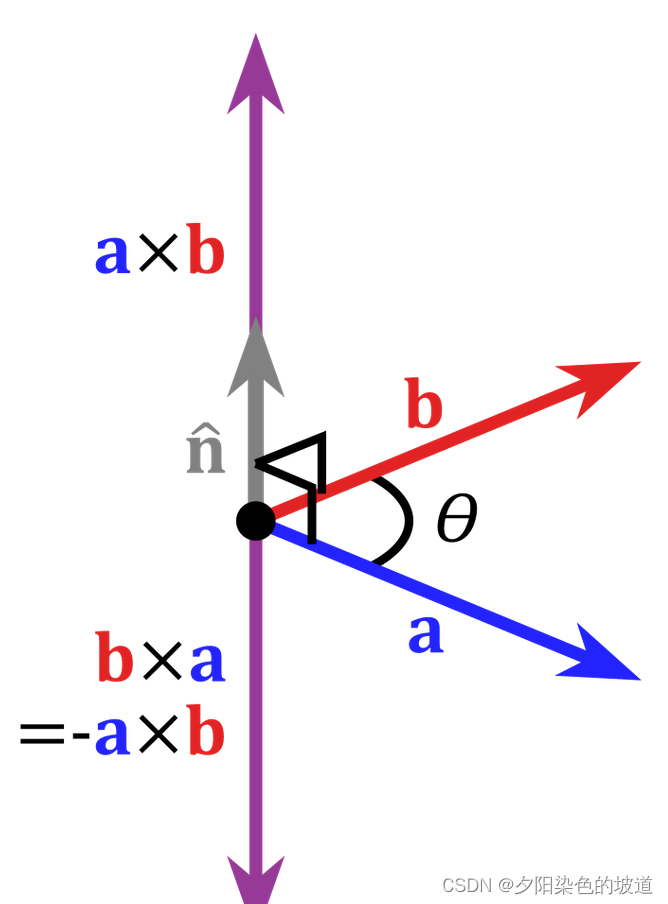上面的定义很好理解。但是一般在代数计算两个向量的叉乘，会用到行列式计算。就如一组单位积 ( i → , j → , k → ) (\overrightarrow{i},\overrightarrow{j},\overrightarrow{k}) ;其中 a → = a 0 i → + a 1 j → + a 2 k → \overrightarrow{a}=a_0\overrightarrow{i}+a_1\overrightarrow{j}+a_2\overrightarrow{k} ; b → = b 0 i → + b 1 j → + b 2 k → \overrightarrow{b}=b_0\overrightarrow{i}+b_1\overrightarrow{j}+b_2\overrightarrow{k}
在计算两个向量的叉乘时候，一般用代数方法为：
a → × b → = ( a 0 i → + a 1 j → + a 2 k → ) × ( b → = b 0 i → + b 1 j → + b 2 k → ) = a 0 b 0 ( i → × i → ) + a 0 b 1 ( i → × j → ) + a 0 b 2 ( i → × k → ) + a 1 b 0 ( j → × i → ) + a 1 b 1 ( j → × j → ) + a 1 b 2 ( j → × k → ) + a 2 b 0 ( k → × i → ) + a 2 b 1 ( k → × j → ) + a 2 b 2 ( k → × k → )      ( 3 ) \overrightarrow{a} \times \overrightarrow{b} = (a_0\overrightarrow{i}+a_1\overrightarrow{j}+a_2\overrightarrow{k}) \times(\overrightarrow{b}=b_0\overrightarrow{i}+b_1\overrightarrow{j}+b_2\overrightarrow{k}) \\ = a_0b_0(\overrightarrow{i} \times \overrightarrow{i}) + a_0b_1(\overrightarrow{i} \times \overrightarrow{j}) + a_0b_2(\overrightarrow{i} \times \overrightarrow{k})+ \\ a_1b_0(\overrightarrow{j} \times \overrightarrow{i}) + a_1b_1(\overrightarrow{j} \times \overrightarrow{j}) + a_1b_2(\overrightarrow{j} \times \overrightarrow{k}) + \\ a_2b_0(\overrightarrow{k} \times \overrightarrow{i}) + a_2b_1(\overrightarrow{k} \times \overrightarrow{j}) + a_2b_2(\overrightarrow{k} \times \overrightarrow{k}) \space \space \space \space(3)

因为基向量 ( i → , j → , k → ) (\overrightarrow{i},\overrightarrow{j},\overrightarrow{k}) 两两垂直，且为单位向量。 0 → \overrightarrow{0} 表示都为 0 0 的向量。所以得到：
i → × i → = 0 →      ( 4 ) j → × j → = 0 →      ( 5 ) k → × k → = 0 →      ( 6 ) i → × j → = k →      ( 7 ) j → × k → = i →      ( 8 ) k → × i → = j →      ( 9 ) \overrightarrow{i} \times \overrightarrow{i}= \overrightarrow{0} \space \space \space \space(4) \\ \overrightarrow{j} \times \overrightarrow{j}= \overrightarrow{0} \space \space \space \space(5) \\ \overrightarrow{k} \times \overrightarrow{k}=\overrightarrow{0} \space \space \space \space(6) \\ \overrightarrow{i} \times \overrightarrow{j}= \overrightarrow{k} \space \space \space \space(7) \\ \overrightarrow{j} \times \overrightarrow{k}= \overrightarrow{i} \space \space \space \space(8)\\ \overrightarrow{k} \times \overrightarrow{i}= \overrightarrow{j} \space \space \space \space(9)
( 4 ) ( 5 ) ( 6 ) ( 7 ) ( 8 ) ( 9 ) (4)(5)(6)(7)(8)(9) 代入公式 ( 3 ) (3) 得到如下：
a → × b → = − a 0 b 0 0 → + a 0 b 1 k → − a 0 b 2 j → − a 1 b 0 k → − a 1 b 1 0 → + a 1 b 2 i → + a 2 b 0 j → − a 2 b 1 i → − a 2 b 2 0 → = ( a 1 b 2 − a 2 b 1 ) i → + ( a 2 b 0 − a 0 b 2 ) j → + ( a 0 b 1 − a 1 b 0 ) k →      ( 10 ) \overrightarrow{a} \times \overrightarrow{b} = -a_0b_0\overrightarrow{0}+a_0b_1\overrightarrow{k}-a_0b_2\overrightarrow{j} \\ - a_1b_0\overrightarrow{k}-a_1b_1\overrightarrow{0} +a_1b_2\overrightarrow{i} \\ +a_2b_0 \overrightarrow{j} - a_2b_1\overrightarrow{i} -a_2b_2\overrightarrow{0}\\ =(a_1b_2-a_2b_1)\overrightarrow{i} + (a_2b_0-a_0b_2)\overrightarrow{j} +(a_0b_1-a_1b_0)\overrightarrow{k} \space \space \space \space(10)

公式的 ( 10 ) (10) ，在日常用行列式计算表达。使用 ( i → , j → , k → ) (\overrightarrow{i},\overrightarrow{j},\overrightarrow{k}) 的矩阵余子式计算方式。它和代数计算方式相等。
a → × b → = [ i → j → k → a 0 a 1 a 2 b 0 b 1 b 2 ] = ( a 1 b 2 − a 2 b 1 ) i → + ( a 2 b 0 − a 0 b 2 ) j → + ( a 0 b 1 − a 1 b 0 ) k → \overrightarrow{a} \times \overrightarrow{b} =\begin{bmatrix} \overrightarrow{i} & \overrightarrow{j} & \overrightarrow{k} \\ a_0& a_1 & a_2 \\ b_0& b_1 & b_2 \end{bmatrix} = (a_1b_2-a_2b_1)\overrightarrow{i} + (a_2b_0-a_0b_2)\overrightarrow{j} +(a_0b_1-a_1b_0)\overrightarrow{k}

因为它为基向量，在欧式几何中，它的表达为：
i → = [ 1 0 0 ] ; j → = [ 0 1 0 ] ; k → = [ 0 0 1 ]      ( 11 ) \overrightarrow{i}=\begin{bmatrix} 1 \\ 0 \\ 0 \end{bmatrix}; \overrightarrow{j}=\begin{bmatrix} 0 \\ 1 \\ 0 \end{bmatrix};\overrightarrow{k}=\begin{bmatrix} 0 \\ 0 \\ 1 \end{bmatrix} \space \space \space \space(11)
因此 ( 11 ) (11) 代入到 ( 10 ) (10) 得到：
a → × b → = [ a 1 b 2 − a 2 b 1 a 2 b 0 − a 0 b 2 a 0 b 1 − a 1 b 0 ]      ( 12 ) \overrightarrow{a} \times \overrightarrow{b} = \begin{bmatrix} a_1b_2-a_2b_1 \\ a_2b_0-a_0b_2 \\ a_0b_1-a_1b_0 \end{bmatrix} \space \space \space \space(12)

上面是基于基向量的表达，它和上面的公式对应，因此可以得到：
a → × b → = ∣ a → ∣ ∣ b → ∣ s i n ( θ ) n → = [ a 1 b 2 − a 2 b 1 a 2 b 0 − a 0 b 2 a 0 b 1 − a 1 b 0 ]      ( 13 ) \overrightarrow{a} \times \overrightarrow{b} = |\overrightarrow{a}||\overrightarrow{b}|sin(\theta)\overrightarrow{n} =\begin{bmatrix} a_1b_2-a_2b_1 \\ a_2b_0-a_0b_2 \\ a_0b_1-a_1b_0 \end{bmatrix} \space \space \space \space(13)

在一些应用，经常向量的表示转化为矩阵的运算。因此(13)公式可以表示矩阵和向量的乘法。
a → × b → = ∣ a → ∣ ∣ b → ∣ s i n ( θ ) n → = [ a 1 b 2 − a 2 b 1 a 2 b 0 − a 0 b 2 a 0 b 1 − a 1 b 0 ] = [ 0 − a 2 a 1 a 2 0 − a 0 − a 1 a 0 0 ] [ b 0 b 1 b 2 ] = a → × b →      ( 14 ) \overrightarrow{a} \times \overrightarrow{b} = |\overrightarrow{a}||\overrightarrow{b}|sin(\theta)\overrightarrow{n} =\begin{bmatrix} a_1b_2-a_2b_1 \\ a_2b_0-a_0b_2 \\ a_0b_1-a_1b_0 \end{bmatrix} = \begin{bmatrix} 0 & -a_2 & a_1 \\ a_2& 0 & -a_0 \\ -a_1& a_0 & 0 \end{bmatrix} \begin{bmatrix} b_0 \\ b_1 \\ b_2 \end{bmatrix} = \overrightarrow{a} \times \overrightarrow{b} \space \space \space \space(14)

两个向量的叉乘仅仅在三维空间有定义。在二维空间没有定义。

下面介绍向量的行列式和向量组成的平行四边形面积的关系。
假设 a → , b → \overrightarrow{a} ,\overrightarrow{b} 为二维向量。这样易于解释。因此画图如下：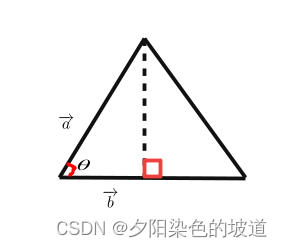计算三角形面积为：
∣ a r e a ∣ = 1 2 ∣ a → ∣ ∣ b → ∣ s i n ( θ )      ( 15 ) |area|=\cfrac{1}{2}|\overrightarrow{a}||\overrightarrow{b}|sin(\theta) \space \space \space \space(15)

转化一下表达，因为 s i n ( θ ) sin(\theta) 不好计算，需要计算 c o s ( θ ) cos(\theta)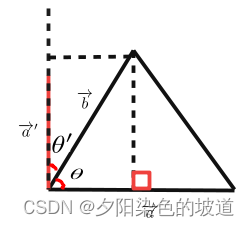其中 ∣ a → ′ ∣ = ∣ a → ∣ |\overrightarrow{a}'|=|\overrightarrow{a}| ; ∣ b → ∣ s i n ( θ ) = ∣ b → ′ ∣ c o s ( θ ′ ) |\overrightarrow{b}|sin(\theta)=|\overrightarrow{b}'|cos(\theta') ;

∣ a r e a ∣ = 1 2 ∣ a → ∣ ∣ b → ∣ s i n ( θ ) = 1 2 ∣ b → ∣ ∣ a → ∣ c o s ( θ ′ )      ( 16 ) |area|=\cfrac{1}{2}|\overrightarrow{a}||\overrightarrow{b}|sin(\theta)=\cfrac{1}{2}|\overrightarrow{b}||\overrightarrow{a}|cos(\theta') \space \space \space \space(16)
其中 θ ′ + θ = 90 \theta'+\theta=90 .且 ∣ a → ′ ∣ = ∣ a → ∣ |\overrightarrow{a}'|=|\overrightarrow{a}| ,容易得到公式简化，简化上述等式为：
∣ a r e a ∣ = 1 2 ∣ b → ∣ ∣ a → ′ ∣ c o s ( θ ′ ) = 1 2 b → ⋅ a → ′ = 1 2 a → ′ ⋅ b →      ( 17 ) |area|=\cfrac{1}{2}|\overrightarrow{b}||\overrightarrow{a}'|cos(\theta')=\cfrac{1}{2}\overrightarrow{b} \cdot \overrightarrow{a}'=\cfrac{1}{2}\overrightarrow{a}' \cdot \overrightarrow{b} \space \space \space \space(17)

因为 a → ′ \overrightarrow{a}' 是通过 a → \overrightarrow{a} 旋转90度得到的，如下图。因此假设 a → = [ a 0 a 1 ] \overrightarrow{a}=\begin{bmatrix} a_0 \\ a_1 \end{bmatrix} 得到 a → ′ = [ − a 1 a 0 ] \overrightarrow{a}'=\begin{bmatrix} -a_1 \\ a_0 \end{bmatrix}

因此得到公式：
2 ∣ a r e a ∣ = a → ′ ⋅ b → = [ − a 1 a 0 ] ⋅ [ b 0 b 1 ] = a 0 b 1 − a 1 b 0      ( 18 ) 2|area|=\overrightarrow{a}' \cdot \overrightarrow{b}=\begin{bmatrix} -a_1 \\ a_0 \end{bmatrix} \cdot \begin{bmatrix} b_0 \\ b_1 \end{bmatrix} = a_0b_1-a_1b_0 \space \space \space \space(18)

可以看到行列式是面积的表达。
2 ∣ a r e a ∣ = ∣ a 0 a 1 b 0 b 1 ∣ 2|area|=\begin{vmatrix} a_0 & a_1 \\ b_0 & b_1 \end{vmatrix}

展开全文机器学习 人工智能
• ## 向量的内、外积及其几何含义

万次阅读 多人点赞 2019-03-18 15:37:51
一、向量的内（点乘） 定义 概括地说，向量的内（点乘/数量）。对两个向量执行点乘运算，就是对这两个向量对应位一一相乘之后求和的操作，如下所示，对于向量a和向量b： a和b的点公式为： 这里要求...

# 一、向量的内积（点乘）

## 定义

概括地说，向量的内积（点乘/数量积）。对两个向量执行点乘运算，就是对这两个向量对应位一一相乘之后求和的操作，如下所示，对于向量a和向量b：a和b的点积公式为：这里要求一维向量a和向量b的行列数相同。注意：点乘的结果是一个标量(数量而不是向量)

定义：两个向量ab的内积为 a·b = |a||b|cos∠(a, b)，特别地，0·a =a·0 = 0；若ab是非零向量，则ab****正交的充要条件是a·b = 0。

## 向量内积的性质：

1. a^2 ≥ 0；当a^2 = 0时，必有a = 0. （正定性）
2. a·b = b·a. （对称性）
3. a + μbc = λa·c + μb·c，对任意实数λ, μ成立. （线性）
4. cos∠(a,b) =a·b/(|a||b|).
5. |a·b| ≤ |a||b|，等号只在ab共线时成立.

## 向量内积的几何意义

内积（点乘）的几何意义包括：

1. 表征或计算两个向量之间的夹角
2. b向量在a向量方向上的投影

有公式：推导过程如下，首先看一下向量组成：定义向量c根据三角形余弦定理（这里a、b、c均为向量，下同）有：根据关系c=a-b有：即：

a∙b=|a||b|cos⁡(θ)

向量a，b的长度都是可以计算的已知量，从而有a和b间的夹角θ：

θ=arccos⁡(a∙b|a||b|)

进而可以进一步判断两个向量是否同一方向或正交(即垂直)等方向关系，具体对应关系为：

a∙b>0→方向基本相同，夹角在0°到90°之间
a∙b=0→ 正交，相互垂直
a∙b<0→ 方向基本相反，夹角在90°到180°之间

# 二、向量的外积（叉乘）

## 定义

概括地说，两个向量的外积，又叫叉乘、叉积向量积，其运算结果是一个向量而不是一个标量。并且两个向量的外积与这两个向量组成的坐标平面垂直。

定义：向量ab的外积a×b是一个向量，其长度等于|a×b| = |a||b|sin∠(a,b)，其方向正交于ab。并且，(a,b,a×b)构成右手系。
特别地，0×a = a×0 = 0.此外，对任意向量aa×a=0

对于向量a和向量b：a和b的外积公式为：其中：根据i、j、k间关系，有：## 向量外积的性质

1. a × b = -b × a. （反称性）
2. a + μb) × c = λ(a ×c) + μ(b ×c). （线性）

## 向量外积的几何意义

在三维几何中，向量a和向量b的外积结果是一个向量，有个更通俗易懂的叫法是法向量，该向量垂直于a和b向量构成的平面。

在3D图像学中，外积的概念非常有用，可以通过两个向量的外积，生成第三个垂直于a，b的法向量，从而构建X、Y、Z坐标系。如下图所示：在二维空间中，外积还有另外一个几何意义就是：|a×b|在数值上等于由向量a和向量b构成的平行四边形的面积。

展开全文• 向量是由n个实数组成的一个n行1...向量的点乘,也叫向量的内、数量，对两个向量执行点乘运算，就是对这两个向量对应位一一相乘之后求和的操作，点乘的结果是一个标量。 点乘公式 对于向量a和向量b： ...

向量是由n个实数组成的一个n行1列（n*1）或一个1行n列（1*n）的有序数组；

向量的点乘,也叫向量的内积、数量积，对两个向量执行点乘运算，就是对这两个向量对应位一一相乘之后求和的操作，点乘的结果是一个标量

## 点乘公式

对于向量a和向量b：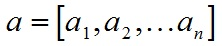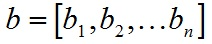a和b的点积公式为：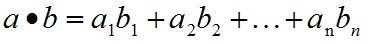要求一维向量a和向量b的行列数相同。

## 点乘几何意义

点乘的几何意义是可以用来表征或计算两个向量之间的夹角，以及在b向量在a向量方向上的投影，有公式：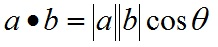推导过程如下，首先看一下向量组成：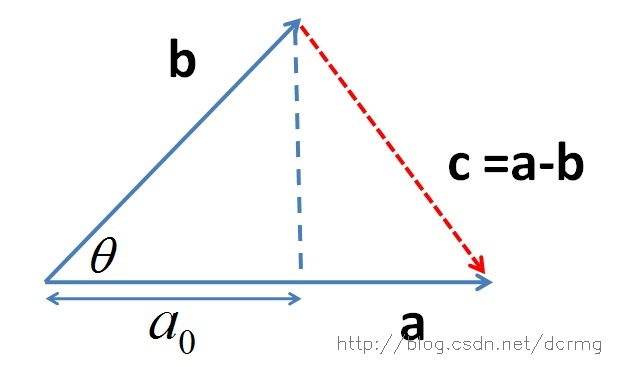定义向量：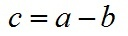根据三角形余弦定理有：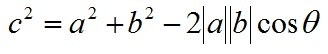根据关系c=a-b（a、b、c均为向量）有：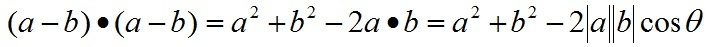即：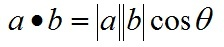向量a，b的长度都是可以计算的已知量，从而有a和b间的夹角θ：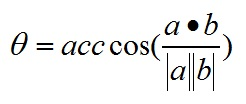根据这个公式就可以计算向量a和向量b之间的夹角。从而就可以进一步判断这两个向量是否是同一方向，是否正交(也就是垂直)等方向关系，具体对应关系为：

a·b>0    方向基本相同，夹角在0°到90°之间

a·b=0    正交，相互垂直

a·b<0    方向基本相反，夹角在90°到180°之间

叉乘公式

两个向量的叉乘，又叫向量积、外积、叉积，叉乘的运算结果是一个向量而不是一个标量。并且两个向量的叉积与这两个向量组成的坐标平面垂直。

对于向量a和向量b：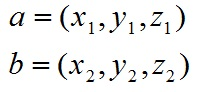a和b的叉乘公式为：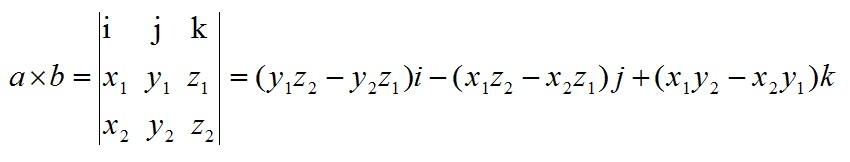其中：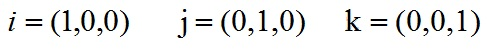根据i、j、k间关系，有：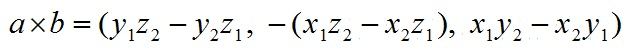## 叉乘几何意义

在三维几何中，向量a和向量b的叉乘结果是一个向量，更为熟知的叫法是法向量，该向量垂直于a和b向量构成的平面。

在3D图像学中，叉乘的概念非常有用，可以通过两个向量的叉乘，生成第三个垂直于a，b的法向量，从而构建X、Y、Z坐标系。如下图所示：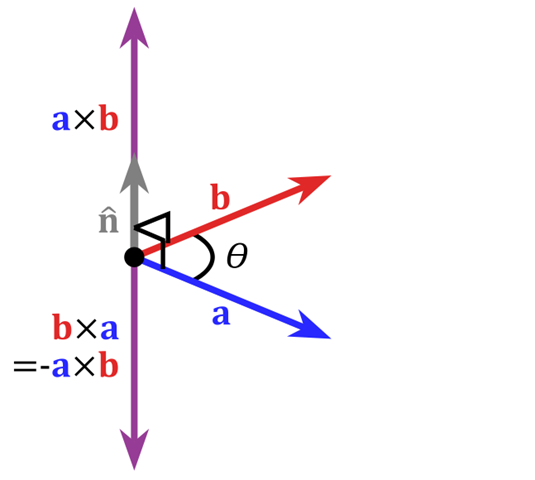在二维空间中，叉乘还有另外一个几何意义就是：aXb等于由向量a和向量b构成的平行四边形的面积。

版权声明：本文为博主原创文章，转载请注明出处。 https://blog.csdn.net/dcrmg/article/details/52416832

===================================================

说是矩阵的叉乘，其实是说的是两个向量的叉乘，矩阵是不能叉乘的。cross(A,B)返回向量A和B的叉乘，其中A，B必须是3个元素的向量！
比如
a=[1,2,3],b=[4,5,6],
则cross(a,b)=[-3 6 -3].
它表示的意思是三维空间中的两个点A(1,2,3)和B(4,5,6),再加上原点O，则构成的两个向量OA，OB，则cross(a,b)就是垂直平面OAB的向量，它的模是三角形OAB面积的2倍。结合上面的例子，假若点C(-3,6,-3),则向量OC就是平面OAB的法向量，|OC|就是三角形OAB面积的2倍。

---------------------
作者：Tiger-Li
来源：CSDN
原文：https://blog.csdn.net/kebu12345678/article/details/80724336
版权声明：本文为作者原创文章，转载请附上博文链接！

展开全文• 向量向量点乘，得到的结果是一个标量，大小等于的与的的乘积与其夹角的余弦 由上可见，其符号由决定,即角的大小定。 几何方向 > 0 主要指向同一方向 = 0 = ...游戏程序
•python
• 向量向量的大小，记作：|向量|。 向量的计算： 向量的各分量的平方和，再开平方。 零向量为0的向量，记作：θ，它的方向任意。 单位向量为1的向量。 负向量： 方向与某向量相反的向量...点积和叉积的区别
• ## 向量的点乘与叉乘的几何意义

万次阅读 多人点赞 2016-11-06 21:59:35
向量的点乘与叉乘的几何意义　很惭愧，作为一名学生，向量的最基本的知识全忘了，在最近做计算机图形学实验时，需要用到向量计算时，发现自己寸步难行。只好赶快百度”预习”一下。向量的点乘:a * b公式：a * b = |a...图形
• 仅在三维空间，两个向量的叉积才有定义，记作 u ^ v ...其中，θ表示u 和 v 的夹角， ||u|| 和 ||v|| 分别是向量 u和v 的，n 则是u、v 所构成平面的法向（垂直于u、v平面的单位向量），方向由右手定则决定。
• 在3D游戏开发中，经常用到向量的点和叉积及其几何意义，为防止遗忘，在此记录一下。点在数学中，点(德语：Skalarprodukt、英语：Dot Product)又称数量或标量(德语：Skalarprodukt、英语：Scalar Product)...
•   向量之间的叉乘和点乘，概念易混淆，分别不清楚，因此本文专门对这个概念进行了详细分析介绍。机器学习
• 一、两向量的数量   数量： a⋅b=∣a∣∣b∣cos⁡θ,a⋅a=∣a∣2\boldsymbol a \cdot \boldsymbol b=|\boldsymbol a||\boldsymbol b| \cos \theta, \quad \boldsymbol a \cdot \boldsymbol a=|\boldsymbol a|^...高等数学
• 几何意义：一个向量长度和它在另一个向量投影长度的乘积。 叉乘、向量积 叉乘是向量积,记作a×b,a×b=｜a｜·｜b｜sinθ,其中｜a｜、｜b｜是两向量的,θ是两向量之间的夹角(0≤θ≤π).以上a与b均为向量。 |向量c|...
•  它的几何意义就是 a 的长度与 b 在 a 上的投影长度的乘积，或者是 b 的长度与 a 在 b 上投影长的乘积，它是一个标量，而 且可正可负。因此互相垂直的向量的内为0。 5、向量的矢（叉积）: a x b ...图形
• 以下说明点乘的几何意义，就是一个向量在另一个单位向量（如果另一个向量是单位向量）上的投影长度。 a * b / |b| = |a|cosθ a * b /|a| = |b|cosθ 2.叉乘的几何意义 [a, b, c]×[d,e,f] = [bf - ec, dc - af, ae ...
• 叉乘（cross product）相对于点乘，叉乘可能更有用吧。...在二维空间里，让我们暂时忽略它的方向，将结果看成一个向量，那么这个结果类似于上述的点，我们有： A x B = |A||B|Sin(θ)然而角度 θ...
• 特征值与特征向量几何意义（转） 2016年9月9日 ReidHolmes Comments 0 Comment   矩阵的乘法是什么，别只告诉我只是“前一个矩阵的行乘以后一个矩阵的列”，还会一点的可能还会说“前一个矩阵的列数...
• 点击上方蓝字关注无距...则A和B的点由如下公式给出：点几何意义是一个向量在另一个向量上的投影。AB可以理解A在B上的投影与B相乘，也可理解为B在A上的投影与A相乘。向量的点也叫数量，两个向量做点得到...matlab 向量 matlab向量的模
• 向量积，数学中又称外积、叉积，物理中称矢积、叉乘，是一种在向量空间中向量的二元运算。与点积不同，它的运算结果是一个向量而不是一个标量。并且两个向量的叉积与这两个向量和垂直。其应用也十分广泛，通常应用于...
• 几何图示： 设有 a=(ax,ay,az),b=(bx,by,bz) \mathbf{a}=\left(a_{x}, a_{y}, a_{z}\right), \mathbf{b}=\left(b_{x}, b_{y}, b_{z}\right) a=(ax​,ay​,az​),b=(bx​,by​,bz​) i，j，k分别是X，Y，Z轴方向的...
• 平面向量的数量平面向量数量的定义已知两个非零向量a和b，它们的夹角为θ，把数量|a||b|cosθ叫做a和b的数量(或内)，记作a·b.即a&m...化和差，指初等数学三角函数部分的一组恒等式。可以通过展开角的...向量数量积公式
• ## 向量的内积和外积

千次阅读 2019-11-11 13:23:43
1 向量，向量的，数量积（内积，点积），向量积（外积，差积） 向量的积有2种： 数量积（也叫内积，点积），是数量，是实数 向量积（也叫外积，差积），是向量 别名这么多，烦它，特此整理一下。 1.1 向量的概念 ...
• 根据内几何意义:一个向量 b 点乘另一个向量 a1 ，等于 b 在 a1 上投影长与 a1 的长的乘积。那我们要求 b 在 a 上的投影长，就用它点乘 a 的单位向量 a1 就可以了，因为单位向量的长度为1， b 的投影长/ c /乘上...图形
• §7.4 数量积 向量积 混合积 一 两向量的数量积 1 向量的数量积定义 设物体在常力的作用下沿直线从点移到点，用表示位移向量，力在位移方向上的分力大小为，力所作的功为： 抛开这一问题的物理背景，我们...高等数学 空间解析几何
• 原文地址 利用向量积（叉积）计算三角形的面积和多边形的面积： 向量的数量积和向量积： （1）向量的数量积 （1）向量的向量积 ...两个向量a和b的叉积（向量积）可以被...求三角形ABC的面积，根据向量积意义，...
• 在物理学和工程学中，几何向量更常被称为矢量。 数量(内、点) 定义：已知两个非零向量a,b，作OA=a,OB=b，则∠AOB称作向量a和向量b的夹角，记作θ并规定0≤θ≤π。 定义：两个向量的数...
• 概念及几何意义解读》相关的范文2011年数学二 考试科目:高等数学.线性代数 考试形式和试卷结构 一.试卷满分及考试时间 试卷满分为150分,考试时间为180分钟． 二.答题方式 答题方式为闭卷.笔试． 三.试卷内容结构 ......